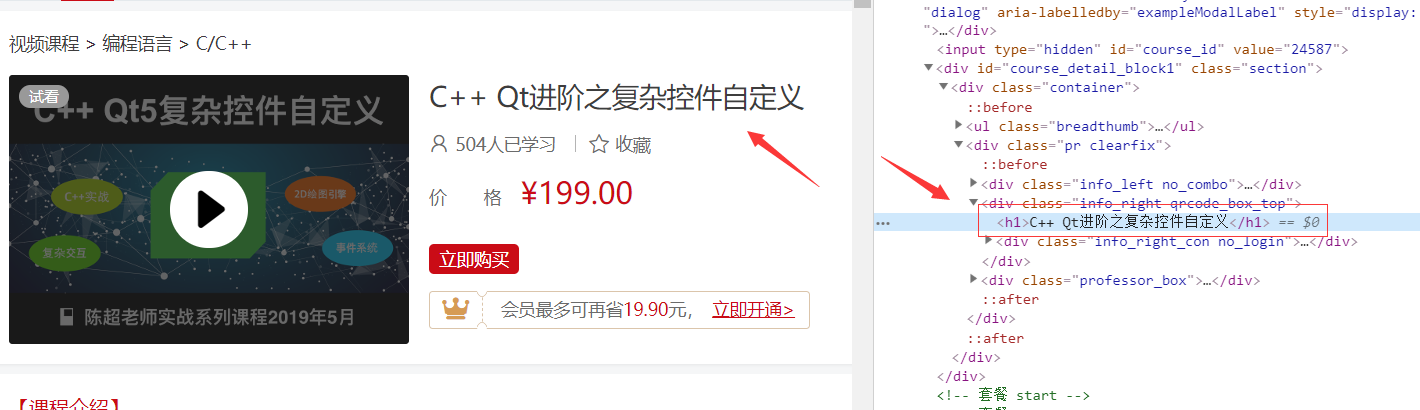Python 正则表达式

通用字符：

\d 匹配数字，0-9
\D 匹配非数字，也就是匹配不是数字的字符
\s 匹配空白符，也就是 空格 \tab
\S 匹配非空白符，跟 \s 相反
\w 匹配数字、字母、下划线， a-z, A-Z, 0-9, _
\W 匹配非数字、字母、下划线

import re

string = 'abcd12345efj'  # 字符串

path = "\w\d\d\d"  # 正则表达式，匹配一个字母后面有3个数字的字符串

resut = re.search(path,string)  # 正则表达式

print(resut)

>>> 输出 <re.Match object; span=(3, 7), match='d123'>

原子表：

import re

string = 'abcd12345efj'  # 字符串

path = "a[bcd]"  # 括号内，满足其一即可

resut = re.search(path,string)

print(resut)

>>> 输出 <re.Match object; span=(0, 2), match='ab'>

ab 满足了括号内其中一个，

import re

string = 'abcd12345efj'  # 字符串

path = "a[^bcd]"  # 除了 ab、ac、ad ，其他的匹配

resut = re.search(path,string)

print(resut)

>>> 输出 <re.Match object; span=(0, 2), match='ab'>

元字符：

.(点) 表示除换行外任意一个字符
^ 如果不在原子表里面，则表示匹配开始位置
\$ 匹配结束位置
* 匹配0次或者多次，可有可无
+ 匹配至少1次
? 匹配1次或者0次，要么有1次，要么没有
{n} 匹配前一个字符出现n次
{n,} 匹配前一个字符至少出现n次
{n,m} 匹配前一个字符出现n到m次
| 模式选择符，表示或
() 模式单元

import re

string = 'abcd12345efj'

path = "a....."

resut = re.search(path,string)

print(resut)

>>> 输出 <re.Match object; span=(0, 6), match='abcd12'>

.(点) 表示除换行外任意一个字符

import re

string = 'abcdddd12345efj'

path = "abcd+"

resut = re.search(path,string)

print(resut)

>>> 输出 <re.Match object; span=(0, 7), match='abcdddd'>

import re

string = 'abcdddd12345efj'

path = "abcd{4}"

resut = re.search(path,string)

print(resut)

>>> 输出 <re.Match object; span=(0, 7), match='abcdddd'>

{4} 表示匹配4个d，包含前面的d

模式修正符：

I 匹配时忽略大小写 （常用）
M 多行匹配 （常用）
L 本地话识别匹配
U unicode
S 让 .(点) 匹配，包括换行符 （常用）

import re

string = 'Python'

path = "py"

resut = re.search(path,string,re.I)

print(resut)

>>> 输出 <re.Match object; span=(0, 2), match='Py'>

resut = re.search(正则表达式,要查找的字符串,模式修饰符)

贪婪模式和懒惰模式：

.* 默认为贪婪模式

import re

string = 'boyboyboy'

path = "b.*y"

resut = re.search(path,string,re.I)

print(resut)

>>> 输出 <re.Match object; span=(0, 9), match='boyboyboy'>

.*? 加问号为懒惰模式

import re

string = 'boyboyboy'

path = "b.*?y"

resut = re.search(path,string,re.I)

print(resut)

>>> 输出 <re.Match object; span=(0, 3), match='boy'>

正则表达式函数：

re.match 从头开始匹配
re.search 任意一个位置都可以匹配
re.compile 全局匹配函数，格式： re.compile(正则表达式).findall(要查找的字符串)
findall 表示为所有

import re

string = '3432bayasdd343boyfghddbsy123'

path = "b.*?y"

resut = re.compile(path,re.I).findall(string)

print(resut)

>>> 输出 ['bay', 'boy', 'bsy']

实例1：匹配 .com 和 .cn 网址

<a href='https://www.baidu.com/'>百度首页</a>

 第三个 **[.com .cn]** 这个就比较好理解了，就是匹配 .com 或者 .cn 的网站

import re

string = "<a href='https://www.baidu.com/'>百度首页</a>"

path = "[a-zA-Z]+://[\S]*[.com|.cn]/"

resut = re.compile(path,re.I).findall(string)

print(resut)

>>> 输出 ['https://www.baidu.com/']

实例2：匹配手机号

\d{3}-\d{8} 就是匹配3位数字的电话号码，比如：010-88888888

import re

string = "abcde010-88888888fghjkl0222-9999999cmbvc"

path = "\d{3}-\d{8}|\d{4}-\d{7}"

resut = re.compile(path,re.I).findall(string)

print(resut)

>>> 输出 ['010-88888888', '0222-9999999']

实例3：简单爬虫编写

urlopen() 函数用来打开 url

import urllib.request

url = 'http://edu.csdn.net'

print(resut)import urllib.request
import re

url = 'https://edu.csdn.net/course/detail/24587?utm_source=banner'

path = '<h1>(.*?)</h1>'

resut = re.compile(path).findall(data)

print(resut)

>>> 输出 ['C++ Qt进阶之复杂控件自定义']